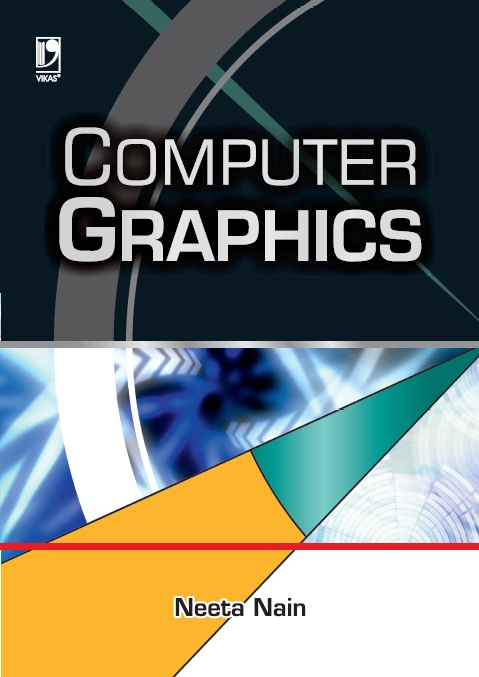# COMPUTER GRAPHICS, 1/eAuthors : NEETA NAIN

• ISBN
• Binding
• Language
• Imprint
• List Price

NEETA NAIN :-
 Neeta Nain has taught computer graphics now for over 20 years. She is a prolific writer having written over 30 research papers for various international journals and conferences, and specialized chapters in three books published by Springer Verlag. The present book, a comprehensive presentation of core mathematical concepts of computer graphics, is a direct result of her vast experience in the subject and intense passion for it.

This book adopts a conceptual approach to computer graphics, with emphasis on mathematical concepts and their applications. It introduces an abstract paradigm that relates the mathematical concepts with computer graphic techniques and implementation methods. This model is intended to help the reader understand the mathematical concepts and their practical use. However, mathematical complexity has not been allowed to dominate. The haul mark of the book is its profuse solved examples which aid in the understanding of mathematical concepts.

The text is supplemented with introduction to various graphics standards, animation, multimedia techniques and fractals. These topics are of immense use in each of the three visual disciplines: modeling transformations, projections and multi-view geometry for computer vision. Geometry of lines, vectors and planes is essential for any geometric computation problem, light and illumination for image-based rendering, and hidden surface removal. Almost every chapter has the working source code to illustrate the concepts, which could be written and used as small programs for better understanding of the topics. A concise appendix of open source OpenGL is also included to showcase programming concepts of computer graphics and visualization.

The text is completely platform-independent and the only prerequisite is the knowledge of coordinate geometry and basic algebra. It will be useful both as a text and reference, thus it can easily be used by novices and experienced practitioners alike.

#### Contents

Introduction to Computer Graphics
• Computer-graphic Primitives
• Scan Conversion
• Transformations
• Projections
• Hidden Surface Removal
• Curves